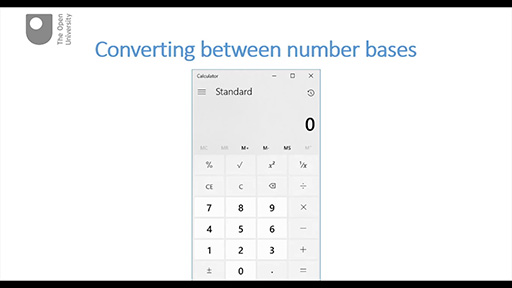Discovering computer networks: hands on in the Open Networking Lab

Start this free course now. Just create an account and sign in. Enrol and complete the course for a free statement of participation or digital badge if available.

Free course

# 7.2 Converting between number bases

This part demonstrates the use of the Windows calculator to convert between binary and decimal number systems and also identifies sources for online tools that will do the same job. There is just one video in this part, which is followed by some questions.

Watch the video below, which is about 4 minutes long.

## Using a calculatorSkip transcript

#### Transcript

Hello. In the previous part we looked at two different number systems: base 10 (decimal) and base 2 (binary). In particular we looked at how large numbers can be represented using both of these number systems. But what do we do if we want to convert a number from one base to another? Fortunately there are tools to help us with this otherwise rather tedious task and I’m going to demonstrate them in this very short part.

The tool I’m going to use is the Windows calculator, but later I’ll identify some online tools you can use instead. You can find the Windows Calculator in Windows Apps.

By default it will open as a standard calculator unless you previously altered the settings, so first of all we need to change the calculator from standard to programmable. Click the Menu button and select Programmer from the list.

Here you select the number base you want to convert from. Mine has opened by default in decimal as you can see from the blue flash at the side. But I can also choose HEX to convert from hexadecimal which uses base 16, OCT to convert from octal which uses base 8, or BIN to convert from binary. I’ll stick with decimal for now.

Let’s say I want to convert the decimal number 10 into binary. I enter the number 10 using the calculator’s keypad, and I’m immediately presented with the hexadecimal, decimal, octal and binary equivalents.

So the binary equivalent of decimal 10 is 1010.

Let’s try a longer decimal number now: how about that number we were working with in the previous part – 3692?

First I’ll need to clear the previous conversion by clicking the C button.

Entering the decimal number 3692 immediately displays the equivalents in the other number bases. So the binary equivalent of decimal 3692 is 1110 0110 1100. Note how longer binary numbers are displayed in groups of four. This makes it easier for us to read them.

Now let’s go the other way from binary to denary. I’ll choose the 8-bit binary number 1010 1010.

First I need to clear the previous conversion by clicking on C and then I need to select the binary input option. Notice how the only symbols I’m offered now are 0 and 1. Now I'll enter the binary number 1010 1010 via the keypad. Immediately the equivalents in the other number bases are displayed.

So the decimal equivalent of binary 1010 1010 is 170. Easy, isn’t it!

There are numerous online converters that you can use to convert between binary, decimal and hex. Here are three that you could try. Or you can find your own by entering ‘number base converter’ into your search engine.

Use the activities that follow this video to experiment with one or more of these online tools and also the Windows Calculator if you have access to it.

End transcript

Interactive feature not available in single page view (see it in standard view).

You’ve seen how you can use the Windows calculator to convert numbers between binary, octal, decimal and hexadecimal number systems. You’ve also been given the links to some online converters.

## Activity 4 Test yourself

5 minutes

• 1. Use a calculator/converter to convert the binary number 10101010 into its decimal equivalent, then use the weighting method of converting from binary to decimal (explained in Section 8.1) to check that you arrive at the same result. (Here you will need to extend your weighting to 28 = 128.)

• The calculator/converter should have given you the answer 170. Using the weighting method your calculations should have been: (1×128) + (0×64) + (1×32) + (0×16) + (1×8) + (0×4) + (1×2) + (0×1) = 128 + 32 + 8 + 2 = 170.

• 2. What is the largest decimal number that could be represented by a 16-symbol binary number? Use a calculator/converter to find out.

• You will need to enter 1111 1111 1111 1111 as the binary number. Your calculator/converter should show you the decimal equivalent 65,535.

• 3. What is the hexadecimal equivalent of the binary number 1111 1111 1111 1111? Use a calculator/converter to find out.

OPNL_1